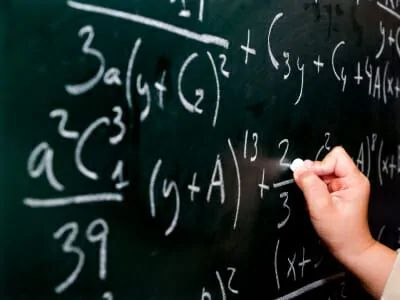## So, What Exactly Is A Maths Degree?

Number crunching: That is the general perception of what a mathematics degree consists of. To the vast majority of the public, they perceive that anyone reading Mathematics at degree level will be able to compute ridiculous sums and gigantic integrals in a mere matter of seconds (In some cases that is true!). But for those brave souls that dare to expand their mathematical potential beyond the scope of A-Level, find an intricate and beautiful world that is engulfed in proofs and equations which seemingly can quantify almost all aspects of life.

The main 4 aspects that Mathematics can be dissected in are Analysis, Algebra, Methods and Applied.

• Analysis is the fundamental building blocks for all of mathematics: Starting only with given definitions and “true” statements, you begin to construct complex yet detailed proofs that explain why certain equations are true.

• Algebra, which in secondary school just meant rearranging equations, is now the wonderful world of groups, rings, fields and other fascinating spaces and algebras. Even with basic linear algebra, you are now able to solve massive linear systems such as simultaneous equations without the need of substitution and cancelling out specific variables.

• Methods is the most similar to mathematics encountered in higher and compulsory education – You have an equation to solve, and you bombard it with all the formulae and techniques you know. What is now different however is that the equations you now deal with have an infinite set of solutions and only when you have certain parameters set, do you in turn get singularly solutions.

• Finally, applied mathematics covers statistics, mechanics as well as computational courses. This area of mathematics is what I deem the most “real” sort of maths, seeing as they can be “applied” (Pardon the pun!) to everything from financial probabilities to river flow dynamics to computer video games.

As a short blog entry, it would be impossible for me to completely describe what an undergraduate maths student would cover over the course of 3 long years. But what I can assure you is that regardless of where  you might plan to take up a maths degree, be prepared for long hours trying to familiarising yourself with the Greek alphabet!

## We Are Here To Help

We have hundreds of tutors available right now to help you improve and succeed. From a one hour session online to a full academic year of face to face lessons, all it takes is five minutes for us to take down your information. We can then find you the most suitable tutors.